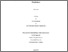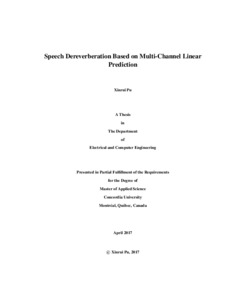Title:

Speech Dereverberation Based on Multi-Channel Linear Prediction

Pu, Xinrui (2017) Speech Dereverberation Based on Multi-Channel Linear Prediction. Masters thesis, Concordia University.Preview
Text (application/pdf)
Pu_MASc_S2017.pdf - Accepted Version
5MB

Abstract

Room reverberation can severely degrade the auditory quality and intelligibility of the speech signals received by distant microphones in an enclosed environment. In recent years, various dereverberation algorithms have been developed to tackle this problem, such as beamforming and inverse filtering of the room transfer function. However, this kind of methods relies heavily on the precise estimation of either the direction of arrival (DOA) or room acoustic characteristics. Thus, their performance is very much limited. A more promising category of dereverberation algorithms has been developed based on multi-channel linear predictor (MCLP). This idea was first proposed in time domain where speech signal is highly correlated in a short period of time. To ensure a good suppression of the reverberation, the prediction filter length is required to be longer than the reverberation time. As a result, the complexity of this algorithm is often unacceptable because of large covariance matrix calculation. To overcome this disadvantage, this thesis focuses on the MCLP dereverberation methods performed in the short-time Fourier transform (STFT) domain.

Recently, the weighted prediction error (WPE) algorithm has been developed and widely applied to speech dereverberation. In WPE algorithm, MCLP is used in the STFT domain to estimate the late reverberation components from previous frames of the reverberant speech. The enhanced speech is obtained by subtracting the late reverberation from the reverberant speech. Each STFT coefficient is assumed to be independent and obeys Gaussian distribution. A maximum likelihood (ML) problem is formulated in each frequency bin to calculate the predictor coefficients. In this thesis, the original WPE algorithm is improved in two aspects. First, two advanced statistical models, generalized Gaussian distribution (GGD) and Laplacian distribution, are employed instead of the classic Gaussian distribution. Both of them are shown to give better modeling of the histogram of the clean speech. Second, we focus on improving the estimation of the variances of the STFT coefficients of the desired signal. In the original WPE algorithm, the variances are estimated in each frequency bin independently without considering the cross-frequency correlation. Thus, we integrate the nonnegative matrix factorization (NMF) into the WPE algorithm to refine the estimation of the variances and hence obtain a better dereverberation performance.

Another category of MCLP based dereverberation algorithm has been proposed in literature by exploiting the sparsity of the STFT coefficients of the desired signal for calculating the predictor coefficients. In this thesis, we also investigate an efficient algorithm based on the maximization of the group sparsity of desired signal using mixed norms. Inspired by the idea of sparse linear predictor (SLP), we propose to include a sparse constraint for the predictor coefficients in order to further improve the dereverberation performance. A weighting parameter is also introduced to achieve a trade-off between the sparsity of the desired signal and the predictor coefficients.

Computer simulation of the proposed dereverberation algorithms is conducted. Our experimental results show that the proposed algorithms can significantly improve the quality of reverberant speech signal under different reverberation times. Subjective evaluation also gives a more intuitive demonstration of the enhanced speech intelligibility. Performance comparison also shows that our algorithms outperform some of the state-of-the-art dereverberation techniques.

Divisions: Concordia University > Gina Cody School of Engineering and Computer Science > Electrical and Computer Engineering Thesis (Masters) Pu, Xinrui Concordia University M.A. Sc. Electrical and Computer Engineering 20 April 2017 Zhu, Wei-Ping 982477 XINRUI PU 09 Jun 2017 14:16 18 Jan 2018 17:55
All items in Spectrum are protected by copyright, with all rights reserved. The use of items is governed by Spectrum's terms of access.

Repository Staff Only: item control page

Back to top